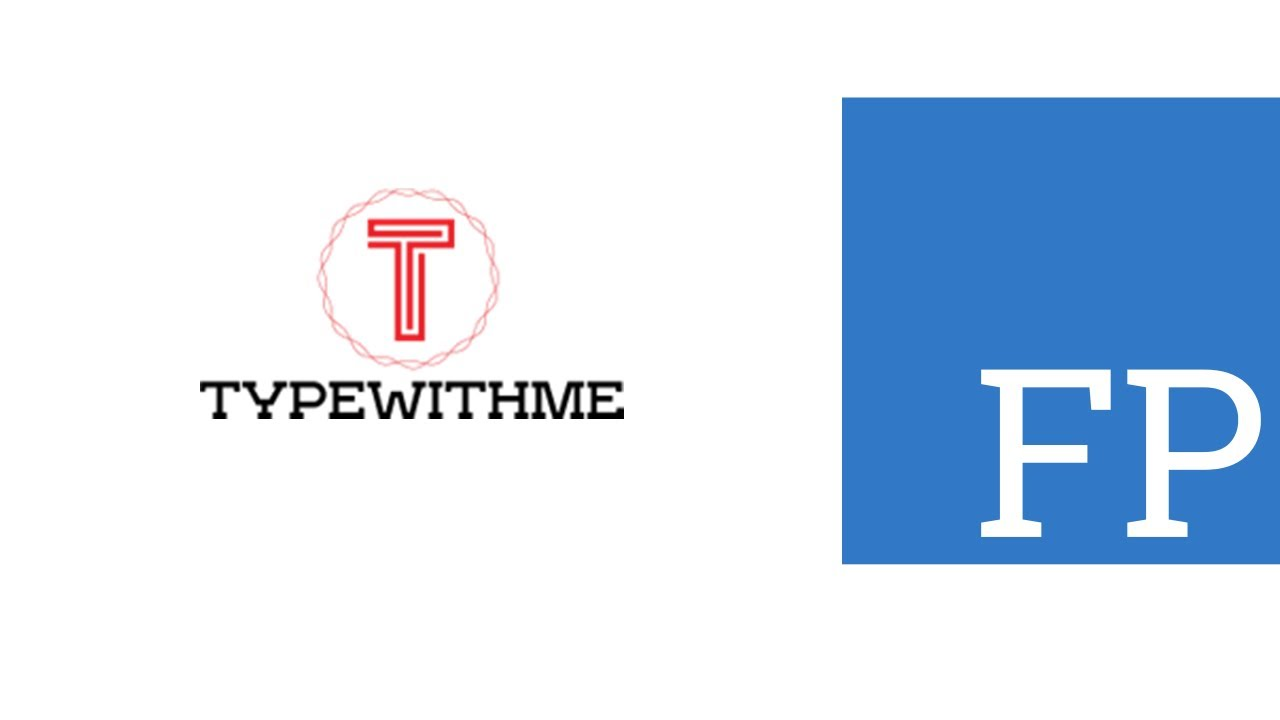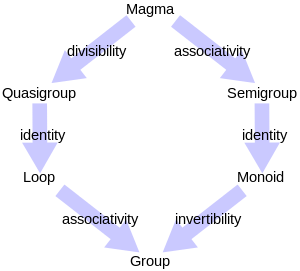1626746880

# Functional Programming with Typescript using Pipe and Flow

Functional programming with typescript using pipe and flow in this first part of a pratical guide of fp-ts, a functional programming library for typescript projects.
You can use pipe and flow to chain a sequence of functions from left-to-right.

What is Fp-ts?
It’s a library that provides abstractions from typed functional languages that makes it easy to apply functional patterns when developing in TypeScript.

This is the first video in a serie of video’s around usefull functions of fp-ts.

#typescript

## Buddha Community1596645240

## Parenthesis Balancing using Monoids in TypeScript

The Parenthesis Balancing problem is a classic problem that says :

Given an expression string exp, write a program to examine whether the pairs and the orders of “(“, “)” are correct in exp.

so this `(()()()())`is balanced but this one `()))` is not

in this article we are going to solve this in a functional manner using Typescript and the monoids from the previous article.

## Monoids in TypeScript

“Alternatively, the fundamental notion of category theory is that of a Monoid”

medium.com

we are going to define a simple balance type that will hold the number of the left end right values.

this amazingly forms a monoid. Since we can concatenate two Balance objects and get a new balance.

#typescript #functional-programming #functional #javascript #monoids #function1596300660

## Functional Programming Series (2): What Is a Monoid?

For those interested in functional programming, I’ll talk about monoids and why they’re very important to understand ahead of time.

Don’t get confused: This isn’t monad — it’s monoid. I’m pretty sure you already know of monoids and you use them almost every day — you just didn’t know the term for them.

# Identity Function

Let’s assume there’s a function named `identity` that takes `A` and returns `A`.

``````const identity: <A>(a: A): A => a;

interface Student {
name: string;
age: number;
}
identity<number>(3) // 3
identity<string>('hello') // hello
identity<Student>({
name: 'Bincent',
age: 5
}); // { name: 'Bincent', age: 5 }
``````

In functional programming, this useless function (seems useless) is an important factor for many other concepts (such as monoids) that we’re about to talk about.Basically, a monoid is a set of elements that holds the rules of the semigroup and the identity-element rule.

If `S` is a set of elements, `a` is a member of `S`, and `·` is a proper binary operation, `a·e = e·a ∈ S` must be satisfied to be a monoid.

``````Identity: a ∈ S, a·e = e·a = a ∈ S
``````

Some documentation calls this using the number 1 and the any alphabet in subscript — for example, 1x referring to the identity on the variable x. Or some documentation uses just a single alphabet letter, such as or e.

That’s all there is to know about monoids, let’s practice with some simple examples.

#typescript #programming #functional-programming #javascript #coding #function1591274700

## Functional Programming: Using the Functor Type Class in TypeScript

This will be a series with a few articles in which I attempt to explain and show the use cases of some functional programming patterns.
We will work with TypeScript and use the functional programming library fp-ts. The examples and explanations are inspired by the great article series by the author of fp-ts.

#programming #typescript #javascript #functional-programming #software-development1595593200

## Functional Programming

Functional Programming is a Declarative style of Programming Paradigm for writing computer programs.
But, What are Functions ?
Functions in general, applies computation on given input and returns the output. It relates input to an output.
f(x) = x + 2;
f(1) = 1 + 2 = 3;
f(2) = 2 + 2 = 4;
Above mentioned is a simple function that adds 2 to the input value and returns output. It relates value [1,2] => [3,4]. Similarly, a function in computer programming is a block of instruction that performs computation on given input and returns the output.
Functional Programming is such a style of writing computer programs using these tiny functions that works together and perform required computation.
Functions in Functional Programming
The philosophy of functional programming is to maintain certain characteristics while writing functions in a computer program. These characteristics are the fundamental nature of functional programming that describe what shall be the behaviour of a function. These are as follows :
Declarative
A function must be declarative, it simply tells what to compute, without specifying how to compute it.
f(x) = x * 4; 👍
Declarative function that tells to multiply input by 4;
f(x) = { y = x + x; z = x + x; return y + z;} 👎
Non-Declarative function that specify how to multiply input by 4;
Pure
A function must give the same output for a given input value, at any period of time. It is not dependent upon anything outside the function definition.
f(x) = It’s never too late; 👍
Pure function that will always return It’s never too late
f(x) = If today’s Friday or Saturday then It’s never too late else It’s late. 👎
Impure function that consider day for returning value. The value is not predictable. It can change. So a function that performs anything which is unpredictable is not a pure function. The condition or the execution is dynamic or unfixed in an impure function.

#development #functional-programming #software #software-programming #declarative-programming #function1623355500

## PANDAS: Most Used Functions in Data Science

### Most useful functions for data preprocessing

When you get introduced to machine learning, the first step is to learn Python and the basic step of learning Python is to learn pandas library. We can install pandas library by pip install pandas. After installing we have to import pandas each time of the running session. The data used for example is from the UCI repository “https://archive.ics.uci.edu/ml/datasets/Heart+failure+clinical+records